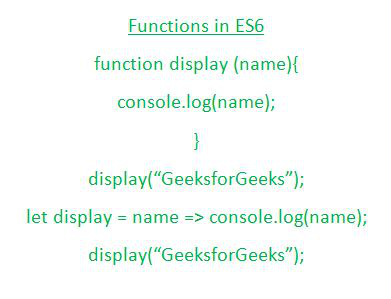# How to define a function in ES6 ?

• Difficulty Level : Expert
• Last Updated : 08 Oct, 2021

In this article, we will try to understand basic details which are associated with the function definition, like syntax declaration of a function or some examples associated with different types of functions declarations in ES6 (EcmaScript-6).

Let us first understand what exactly the function is and how to declare a function in ES6 using different syntaxes.

Hey geek! The constant emerging technologies in the world of web development always keeps the excitement for this subject through the roof. But before you tackle the big projects, we suggest you start by learning the basics. Kickstart your web development journey by learning JS concepts with our JavaScript Course. Now at it's lowest price ever!Functions:

• Functions are one of the basic fundamental blocks in JavaScript.
• It contains those lines of codes that the user wishes to either reuse or just invoke only when that function is invoked.
• A function may take no input parameter or may have a different number of parameters.
• A function must have either a return statement or a print statement which would be executed while that function is invoked at the point during the program execution.
• A function can be declared with different syntaxes, few of them are illustrated in the above pictorial representation.

Following are some of the syntax which can be used to declare a function in ES6:

Syntax 1: The first syntax is the basic syntax that is valid from the starting ES version till this ES6 version as well.

```function function_name (list_of_parameters) {
...
}```

If one wishes to store the function in a variable then by using the following syntax one can easily do that.

```let variable = function (list_of_parameters) {
...
}```

Syntax 2: Now another syntax of declaring a function is the Arrow function syntax which is described as follows and the calling of these particular type of function is quite simple as that of the previous type of functions which is just writing the name followed by the round braces, including the parameters if any (like this display(name) and so on).

```let variable = (list_of_parameters) => {
...
}```

Even if we don’t want to write the round braces then also we may proceed by using the following syntax.

```let variable = parameters => {
...
}```

Now that we have analyzed who writes a function in ES6 let us quote some examples which would help us to understand the functions declarations in a better and effective way.

Example 1: In this example, we will see the normal function declaration.

## Javascript

 ``

Output: The output of the above code snippet is shown below-

```25
196
361```

Example 2: In this example, we will be using Rest parameters inside the normal function (which actually means that we are passing an infinite number of values as parameters of a function) and further checking the length of passed parameters.

## Javascript

 ``

Output: The output of the above code snippet would be as follows-

```3
1
5
2
0```

Example 3: In this, we will using arrow function syntax, and by using that we will be finding out the factorial of the given number.

## Javascript

 ``

Output: The output of the above code snippet would be as follows-

```3628800
121645100408832000
120```

My Personal Notes arrow_drop_up